Next: Displacement Operators Up: Position and Momentum Previous: Momentum Representation

# Heisenberg Uncertainty Principle

How is a momentum space wavefunction related to the corresponding coordinate space wavefunction? To answer this question, let us consider the representative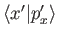of the momentum eigenkets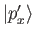in the Schrödinger representation for a system with a single degree of freedom. This representative satisfies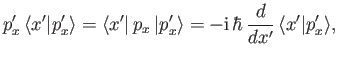(2.91)

where use has been made of Equation (2.78) (for the case of a system with one degree of freedom). The solution of the previous differential equation is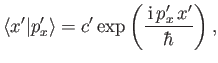(2.92)

where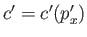. It is easily demonstrated that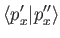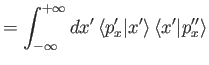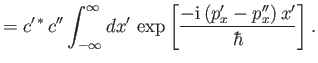(2.93)

The well-known mathematical result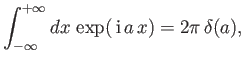(2.94)

yields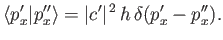(2.95)

This is consistent with Equation (2.80), provided that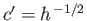. Thus,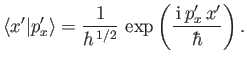(2.96)

Consider a general state ket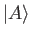whose coordinate wavefunction is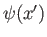, and whose momentum wavefunction is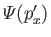. In other words,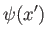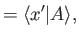(2.97)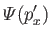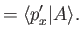(2.98)

It is easily demonstrated that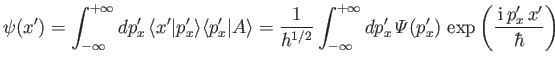(2.99)

and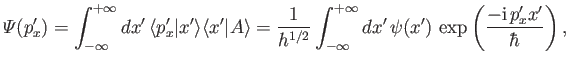(2.100)

where use has been made of Equations (2.27), (2.81), (2.94), and (2.96). Clearly, the momentum space wavefunction is the Fourier transform of the corresponding coordinate space wavefunction .

Consider a state whose coordinate space wavefunction is a wavepacket. In other words, the wavefunction only has non-negligible amplitude in some spatially localized region of extent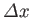. As is well known, the Fourier transform of a wavepacket fills up a wavenumber band of approximate extent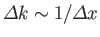. . Note that, in Equation (2.99), the role of the wavenumber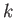is played by the quantity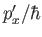. It follows that the momentum space wavefunction corresponding to a wavepacket in coordinate space extends over a range of momenta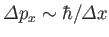. Clearly, a measurement ofis almost certain to give a result lying in a range of width. Likewise, measurement of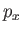is almost certain to yield a result lying in a range of width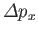. The product of these two uncertainties is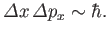(2.101)

This result is called the Heisenberg uncertainty principle .

Actually, it is possible to write the Heisenberg uncertainty principle more exactly by making use of Equation (1.86) and the commutation relation (2.47). We obtain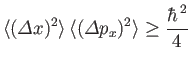(2.102)

for a general state. It is easily demonstrated that the minimum uncertainty states, for which the equality sign holds in the previous relation, correspond to Gaussian wavepackets in both coordinate and momentum space. (See Exercise 4.)Next: Displacement Operators Up: Position and Momentum Previous: Momentum Representation
Richard Fitzpatrick 2016-01-22